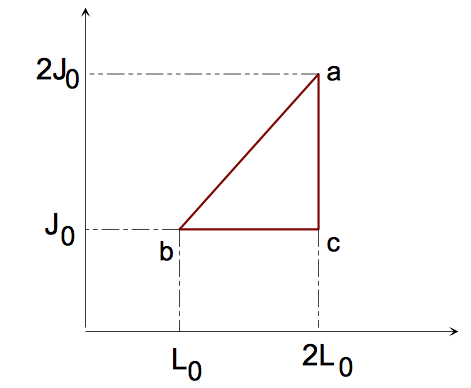# Heat engine that uses a rubber band (Thermodynamics)

Pedro Roman
Homework Statement:
Find the efficiency of a three-step cycle heat engine that uses a rubber band (the cycle is shown in the figure), which idealized equation of state is J= aLT (J is the tension, L is the length of the rubber band per unit mass, a is a constant and T is the temperature). The specific heat capacity is a constant, c. Find the efficiency of this rubber band engine.
Relevant Equations:
dQ=TdS=(dS/dT)dT+(dS/dL)dLNota that the path a to b is isothermal since J is proportional to L, then we can find the value of T_a and T_b using the equation of state and the figure. We have,

\begin{equation}
J_0=\alpha L_0T_b
\end{equation}
or
\begin{equation}
T_b=T_a=\frac{J_0}{\alpha L_0}=T_0
\end{equation}

Also, by using the equation of state we find,

\begin{equation}
T_c=T_0/2
\end{equation}

Now, for the path a to b, we have that

\begin{equation}
\begin{split}
dQ&=T_0dS=T_0\left(\frac{\partial S}{\partial L}\right)_T dL\\
dQ&=-T_0\left(\frac{\partial J}{\partial T}\right)_L dL\\
dQ&=-\alpha LM T_0dL\\
\end{split}
\end{equation}
where M is the mass of the rubber band. Therefore,
\begin{equation}\Delta Q_{ab}=\frac{3L_0J_0M}{2}.\end{equation}

For b to c, we have
\begin{equation}
\begin{split}
dQ=& T\left(\frac{\partial S}{\partial T}\right)_LdT \\
+& T\left(\frac{\partial S}{\partial L}\right)_TdL\\
dQ=&cM dT-\alpha LMT dL\\
dQ=&cM dT-J_0 dL\\
\end{split}
\end{equation}
where I have used the equation of state. Now the change of heat is given by
\begin{equation}\Delta Q_{bc}=-\frac{McT_0}{2}-J_0L_0M.\end{equation}
Finally for c to a we have dL=0 and
\begin{equation}
\begin{split}
dQ=& T\left(\frac{\partial S}{\partial T}\right)_L dT \\
dQ=&cM dT\\
\end{split}
\end{equation}
Then, we find,
\begin{equation}
\Delta Q_{ca}=\frac{McT_0}{2}.
\end{equation}

The total work must be (since for a full cycle dU=0),
\begin{equation}
\begin{split}
\Delta W_{tot}&=\Delta Q_{tot}\\
\Delta W_{tot}&=\Delta Q_{ab}+\Delta Q_{bc}+\Delta Q_{ca}\\
\Delta W_{tot}&=\frac{J_0L_0M}{2}.
\end{split}
\end{equation}
The absorbed heat must be the heat changes that are positive (right?), then,
\begin{equation}
\begin{split}
\Delta Q_{abs}&=\Delta Q_{ab}+\Delta Q_{ca}\\
\Delta Q_{abs}&=\frac{3}{2}J_0L_0M+\frac{McT_0}{2}
\end{split}
\end{equation}
At last, we obtain the efficiency,
\begin{equation}
\begin{split}
\eta &=\frac{\Delta W_{tot}}{\Delta Q_{abs}}\\
\eta &=\frac{1}{3+\frac{cT_0}{J_0L_0}}.
\end{split}
\end{equation}
So, I would thank you if you could tell me if that efficiency is correct, I saw some pages solving the same problem that find the efficiency to be 1/3, so I am not sure about my calculation. Thanks in advance.

Last edited:

•